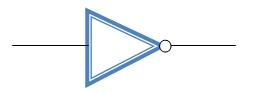## Design Of D-Flip Flop Using Verilog

Inverter is a logic gate, with one input and one output. Its symbol is shown below:-The output of inverter is complement of the input i.e., if the input is 0, the output will be 1 and vice-versa. The truth table for inverter is shown below:-

Input Output
0 1
1 0

The transistor level schematic of inverter can be designed in many logics, following two logics will be used for designing in the experiment

1. Complementary CMOS logic
2. Pseudo NMOS logic